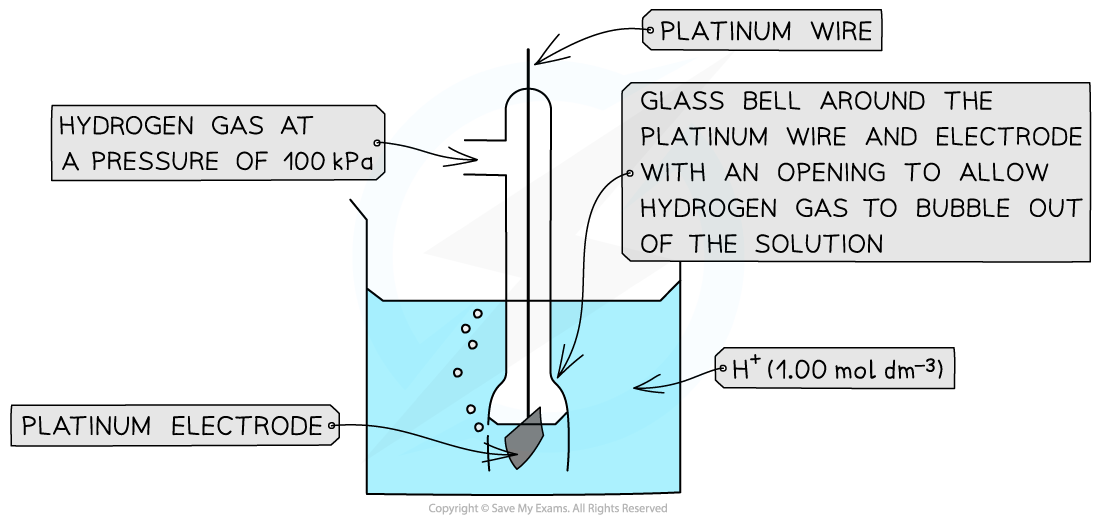# Edexcel A Level Chemistry:复习笔记6.1.2 Standard Electrode Potential

### Standard Electrode Potentials

#### Standard electrode potential

• The position of equilibrium and therefore the electrode potential depends on factors such as:
• Temperature
• Pressure of gases
• Concentration of reagents

• So, to be able to compare the electrode potentials of different species, they all have to be measured against a common reference or standard
• Standard conditions also have to be used when comparing electrode potentials
• These standard conditions are:
• Ion concentration of 1.00 mol dm-3
• A temperature of 298 K
• A pressure of 100 kPa

• Standard measurements are made using a high resistance voltmeter so that no current flows and the maximum potential difference is achieved
• The electrode potentials are measured relative to a standard hydrogen electrode
• The standard hydrogen electrode is given a value of 0.00 V, and all other electrode potentials are compared to this standard
• This means that the electrode potentials are always referred to as a standard electrode potential (Eꝋ)
• The standard electrode potential (Eꝋ) is the potential difference ( sometimes called voltage) produced when a standard half-cell is connected to a standard hydrogen cell under standard conditions
• For example, the standard electrode potential of bromine suggests that relative to the hydrogen half-cell it is more likely to get reduced, as it has a more positive Eꝋ value

Br2(l) + 2e– ⇌ 2Br–(aq)        Eꝋ = +1.09 V

2H+(aq) + 2e– ⇌ H2(g)        Eꝋ = 0.00 V

• The standard electrode potential of sodium, on the other hand, suggests that relative to the hydrogen half-cell it is less likely to get reduced as it has a more negative Eꝋ value

Na+ (aq) + e– ⇌ Na(s)        Eꝋ = -2.71 V

2H+ (aq) + 2e– ⇌ H2(g)        Eꝋ = 0.00 V

### The Standard Hydrogen Electrode, SHE

• The standard hydrogen electrode is a half-cell used as a reference electrode and consists of:
• Hydrogen gas in equilibrium with H+ ions of concentration 1.00 mol dm-3 (at 100 kPa)

2H+ (aq) + 2e- ⇌ H2 (g)

• An inert platinum electrode that is in contact with the hydrogen gas and H+ ions
• When the standard hydrogen electrode is connected to another half-cell, the standard electrode potential of that half-cell can be read off a high resistance voltmeterThe standard electrode potential of a half-cell can be determined by connecting it to a standard hydrogen electrode